# Top 12 Python Libraries for Machine Learning and Data Science in 2020Python has been the go-to choice for Machine Learning, Data Science and Artificial Intelligence developers for a long time. Python libraries for modern machine learning models & projects: TensorFlow; Numpy; Scipy; Scikit-learn; Theano; Keras; PyTorch; Pandas; Matplotlib; ...

Machine Learning and Deep Learning have been on the rise recently with the push in the AI industry and the early adopters of this technology are beginning to see it bear its fruits. As more and more businesses jump into the bandwagon and start investing their time and efforts into realizing the potential of this untapped domain, the better this is going to get for the developers working in the area.

Several programming languages can get you started with AI, ML and DL with each language offering stronghold on a specific concept. Some of the popular programming languages for ML and DL are Python, Julia, R, Java along with a few more. As for now, we’ll be focussing more on Python.

## Why is Python Preferred for Machine Learning and AI?

Python seems to be winning battle as preferred language of MachineLearning. The availability of libraries and open source tools make it ideal choice for developing ML models.

Python has been the go-to choice for Machine Learning and Artificial Intelligence developers for a long time. Python offers some of the best flexibilities and features to developers that not only increase their productivity but the quality of the code as well, not to mention the extensive libraries helping ease the workload. Various features that put Python among the top programming languages for Machine Learning, Deep Learning and Artificial Intelligence are listed below:

Free and open-source nature makes it community friendly and guarantees improvements in the long run

Exhaustive libraries ensure there’s a solution for every existing problem

Smooth implementation and integration make it accessible for people with the varying skill level to adapt it

● Increased productivity by reducing the time to code and debug

● Can be used for Soft Computing, Natural Language Processing as well

Works seamlessly with C and C++ code modules

Python is the most powerful language you can still read. - Pau Dubois

## Best Python Libraries for Machine Learning and Deep Learning

Machine Learning, as the name suggests, is the science of programming a computer by which they are able to learn from different kinds of data. A more general definition given by Arthur Samuel is – “Machine Learning is the field of study that gives computers the ability to learn without being explicitly programmed.” They are typically used to solve various types of life problems. In the older days, people used to perform Machine Learning tasks by manually coding all the algorithms and mathematical and statistical formula. This made the process time consuming, tedious and inefficient. But in the modern days, it is become very much easy and efficient compared to the olden days by various python libraries, frameworks, and modules. Today, Python is one of the most popular programming languages for this task and it has replaced many languages in the industry, one of the reason is its vast collection of libraries.## TensorFlow

The revolution is here! Welcome to TensorFlow 2.0.

TensorFlow is a fast, flexible, and scalable open-source machine learning library for research and production.

TensorFlow is one of the best library available for working with Machine Learning on Python. Offered by Google, TensorFlow makes ML model building easy for beginners and professionals alike.

TensorFlow is a very popular open-source library for high performance numerical computation developed by the Google Brain team in Google. As the name suggests, Tensorflow is a framework that involves defining and running computations involving tensors. It can train and run deep neural networks that can be used to develop several AI applications. TensorFlow is widely used in the field of deep learning research and application.

``````# Python program using TensorFlow
# for multiplying two arrays

# import `tensorflow`
import tensorflow as tf

# Initialize two constants
x1 = tf.constant([1, 2, 3, 4])
x2 = tf.constant([5, 6, 7, 8])

# Multiply
result = tf.multiply(x1, x2)

# Initialize the Session
sess = tf.Session()

# Print the result
print(sess.run(result))

# Close the session
sess.close() ``````

Output:

``[ 5 12 21 32]``

Using TensorFlow, you can create and train ML models on not just computers but also mobile devices and servers by using TensorFlow Lite and TensorFlow Serving that offers the same benefits but for mobile platforms and high-performance servers.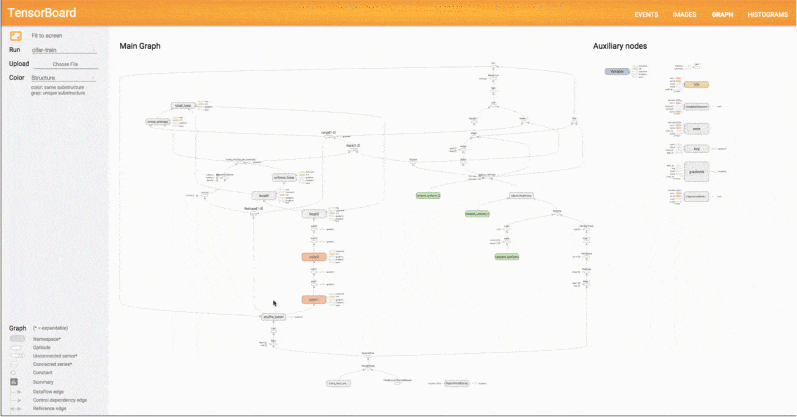Some of the essential areas in ML and DL where TensorFlow shines are:

● Handling deep neural networks

● Natural Language Processing

● Partial Differential Equation

● Abstraction capabilities

● Image, Text, and Speech recognition

● Effortless collaboration of ideas and code

Core Task: Build Deep Learning models

## KerasKerasis one of the most popular and open-source neural network libraries for Python. Initially designed by a Google engineer for ONEIROS, short for Open-Ended Neuro Electronic Intelligent Robot Operating System, Keras was soon supported in TensorFlow’s core library making it accessible on top of TensorFlow.

Keras is a very popular Machine Learning library for Python. It is a high-level neural networks API capable of running on top of TensorFlow, CNTK, or Theano. It can run seamlessly on both CPU and GPU. Keras makes it really for ML beginners to build and design a Neural Network. One of the best thing about Keras is that it allows for easy and fast prototyping.

Keras features several of the building blocks and tools necessary for creating a neural network such as:

● Neural layers

● Activation and cost functions

● Objectives

● Batch normalization

● Dropout

● Pooling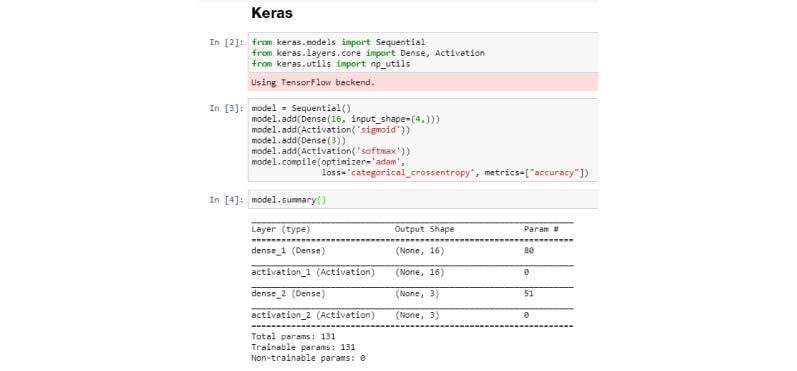Keras extends the usability of TensorFlow with these additional features for ML and DL programming. With a helpful community and a dedicated Slack channel, getting support is easy. Support for the convolutional and recurrent neural network also exists along with standard neural networks. You can also refer to other example models in Keras and Computer Vision class from Stanford.

Core Task: Build Deep Learning models

Getting Started with Keras —

## PyTorch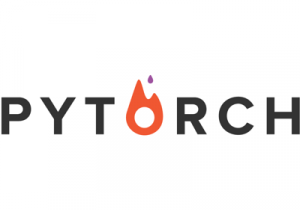Developed by Facebook, PyTorch is one of the few machine learning libraries for Python. Apart from Python, PyTorch also has support for C++ with its C++ interface if you’re into that. Considered among the top contenders in the race of being the best Machine Learning and Deep Learning framework, PyTorch faces touch competition from TensorFlow. You can refer to the PyTorch tutorials for other details.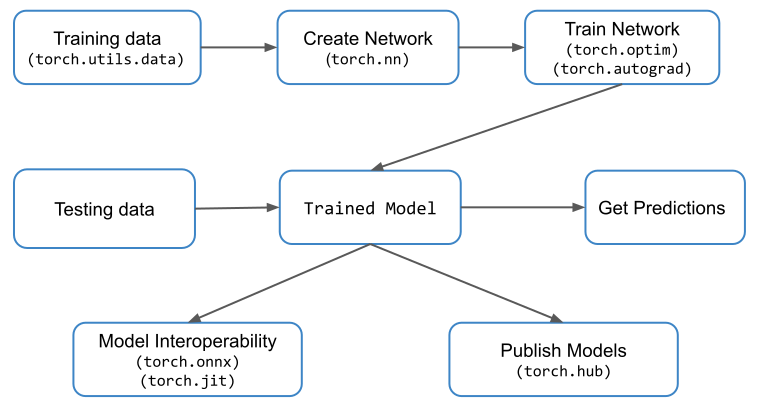PyTorch is a popular open-source Machine Learning library for Python based on Torch, which is an open-source Machine Learning library which is implemented in C with a wrapper in Lua. It has an extensive choice of tools and libraries that supports on Computer Vision, Natural Language Processing(NLP) and many more ML programs. It allows developers to perform computations on Tensors with GPU acceleration and also helps in creating computational graphs.

``````# Python program using PyTorch
# for defining tensors fit a
# two-layer network to random
# data and calculating the loss

import torch

dtype = torch.float
device = torch.device("cpu")
# device = torch.device("cuda:0") Uncomment this to run on GPU

# N is batch size; D_in is input dimension;
# H is hidden dimension; D_out is output dimension.
N, D_in, H, D_out = 64, 1000, 100, 10

# Create random input and output data
x = torch.randn(N, D_in, device = device, dtype = dtype)
y = torch.randn(N, D_out, device = device, dtype = dtype)

# Randomly initialize weights
w1 = torch.randn(D_in, H, device = device, dtype = dtype)
w2 = torch.randn(H, D_out, device = device, dtype = dtype)

learning_rate = 1e-6
for t in range(500):
# Forward pass: compute predicted y
h = x.mm(w1)
h_relu = h.clamp(min = 0)
y_pred = h_relu.mm(w2)

# Compute and print loss
loss = (y_pred - y).pow(2).sum().item()
print(t, loss)

# Backprop to compute gradients of w1 and w2 with respect to loss
grad_y_pred = 2.0 * (y_pred - y)

# Update weights using gradient descent
w2 -= learning_rate * grad_w2 ``````

Output:

``````0 47168344.0
1 46385584.0
2 43153576.0
...
...
...
497 3.987660602433607e-05
498 3.945609932998195e-05
499 3.897604619851336e-05``````

For more details refer to documentation.

Some of the vital features that set PyTorch apart from TensorFlow are:

● Tensor computing with the ability for accelerated processing via Graphics Processing Units

● Easy to learn, use and integrate with the rest of the Python ecosystem

● Support for neural networks built on a tape-based auto diff system

The various modules PyTorch comes with, that help create and train neural networks:

● Tensors — torch.Tensor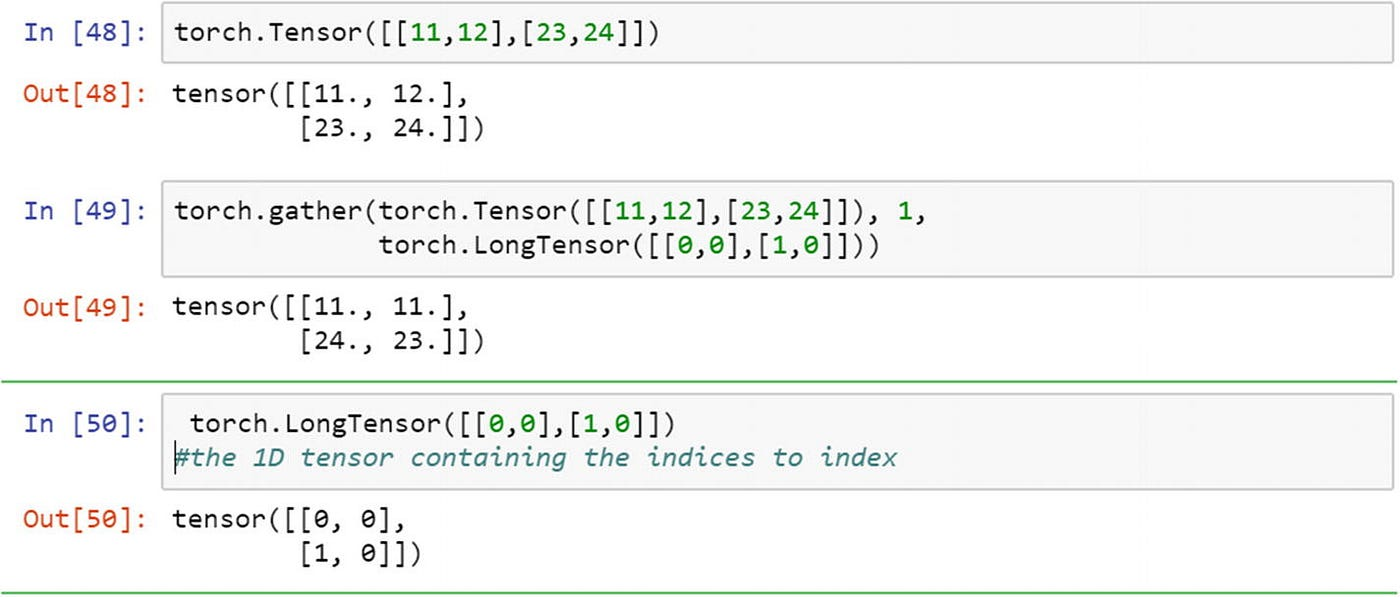● Optimizers — torch.optim module

● Neural Networks — nn module

Pros: very customizable, widely used in deep learning research

Cons: fewer NLP abstractions, not optimized for speed

Core task: Developing and training deep learning models

## Scikit-learnScikit-learn is another actively used machine learning library for Python. It includes easy integration with different ML programming libraries like NumPy and Pandas.

Skikit-learn is one of the most popular ML libraries for classical ML algorithms. It is built on top of two basic Python libraries, viz., NumPy and SciPy. Scikit-learn supports most of the supervised and unsupervised learning algorithms. Scikit-learn can also be used for data-mining and data-analysis, which makes it a great tool who is starting out with ML.

``````# Python script using Scikit-learn
# for Decision Tree Clasifier

# Sample Decision Tree Classifier
from sklearn import datasets
from sklearn import metrics
from sklearn.tree import DecisionTreeClassifier

# fit a CART model to the data
model = DecisionTreeClassifier()
model.fit(dataset.data, dataset.target)
print(model)

# make predictions
expected = dataset.target
predicted = model.predict(dataset.data)

# summarize the fit of the model
print(metrics.classification_report(expected, predicted))
print(metrics.confusion_matrix(expected, predicted)) ``````

Output:

``````DecisionTreeClassifier(class_weight=None, criterion='gini', max_depth=None,
max_features=None, max_leaf_nodes=None,
min_impurity_decrease=0.0, min_impurity_split=None,
min_samples_leaf=1, min_samples_split=2,
min_weight_fraction_leaf=0.0, presort=False, random_state=None,
splitter='best')
precision    recall  f1-score   support

0       1.00      1.00      1.00        50
1       1.00      1.00      1.00        50
2       1.00      1.00      1.00        50

micro avg       1.00      1.00      1.00       150
macro avg       1.00      1.00      1.00       150
weighted avg       1.00      1.00      1.00       150

[[50  0  0]
[ 0 50  0]
[ 0  0 50]]``````

Scikit-learn comes with the support of various algorithms such as:

● Classification

● Regression

● Clustering

● Dimensionality Reduction

● Model Selection

● Preprocessing

Built around the idea of being easy to use but still be flexible, Scikit-learn is focussed on data modelling and not on other tasks such as loading, handling, manipulation and visualization of data. It is considered sufficient enough to be used as an end-to-end ML, from the research phase to the deployment. For a deeper understanding of scikit-learn, you can check out the Scikit-learn tutorials.

Learn Scikit-Learn-

## Pandas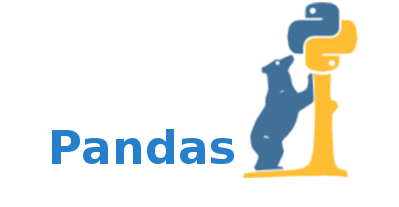Pandas is a Python data analysis library and is used primarily for data manipulation and analysis. It comes into play before the dataset is prepared for training. Pandas make working with time series and structured multidimensional data effortless for machine-learning programmers.

Pandas is a popular Python library for data analysis. It is not directly related to Machine Learning. As we know that the dataset must be prepared before training. In this case, Pandas comes handy as it was developed specifically for data extraction and preparation. It provides high-level data structures and wide variety tools for data analysis. It provides many inbuilt methods for groping, combining and filtering data.

``````# Python program using Pandas for
# arranging a given set of data
# into a table

# importing pandas as pd
import pandas as pd

data = {"country": ["Brazil", "Russia", "India", "China", "South Africa"],
"capital": ["Brasilia", "Moscow", "New Dehli", "Beijing", "Pretoria"],
"area": [8.516, 17.10, 3.286, 9.597, 1.221],
"population": [200.4, 143.5, 1252, 1357, 52.98] }

data_table = pd.DataFrame(data)
print(data_table)
``````

Output: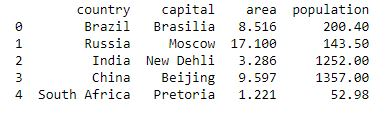Some of the great features of Pandas when it comes to handling data are:

● Dataset reshaping and pivoting

● Merging and joining of datasets

● Handling of missing data and data alignment

● Various indexing options such as Hierarchical axis indexing, Fancy indexing

● Data filtration options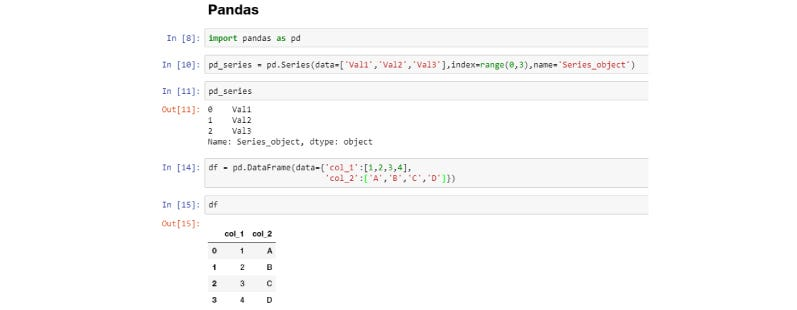Pandas make use of DataFrames, which is just a technical term for a two-dimensional representation of data by offering programmers with DataFrame objects.

Core task: Data manipulation and analysis

Google Trends — Pandas Interest Over Time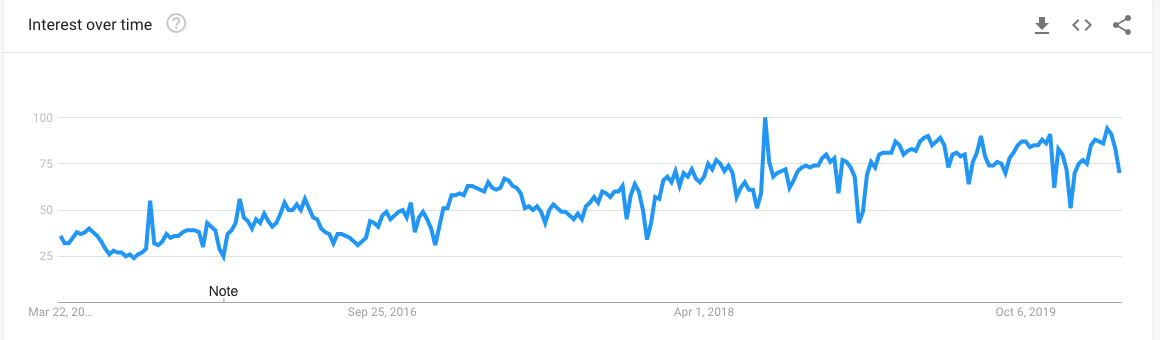## NLTK

NLTK stands for Natural Language Toolkit and is a Python library for working with natural language processing. It is considered as one of the most popular libraries to work with human language data. NLTK offers simple interfaces along with a wide array of lexical resources such as FrameNet, WordNet, Word2Vec and several others to programmers. Some of the highlights of NLTK are:

● Searching keywords in documents

● Tokenization and classification of texts

● Recognition on voice and handwriting

● Lemmatizing and Stemming of words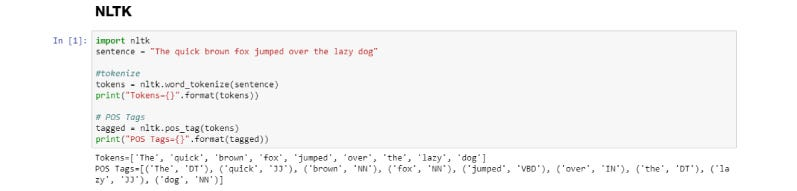NLTK and its suite of packages are considered a reliable choice for students, engineers, researchers, linguists and industries that work with language.

## Spark MLlib

MLlib is Apache Spark’s scalable machine learning library

Developed by Apache, Spark MLlib is a machine learning library that enables easy scaling of your computations. It is simple to use, quick, easy to set up and offers smooth integration with other tools. Spark MLlib instantly became a convenient tool for developing machine learning algorithms and applications.

The tools that Spark MLlib brings to the table are: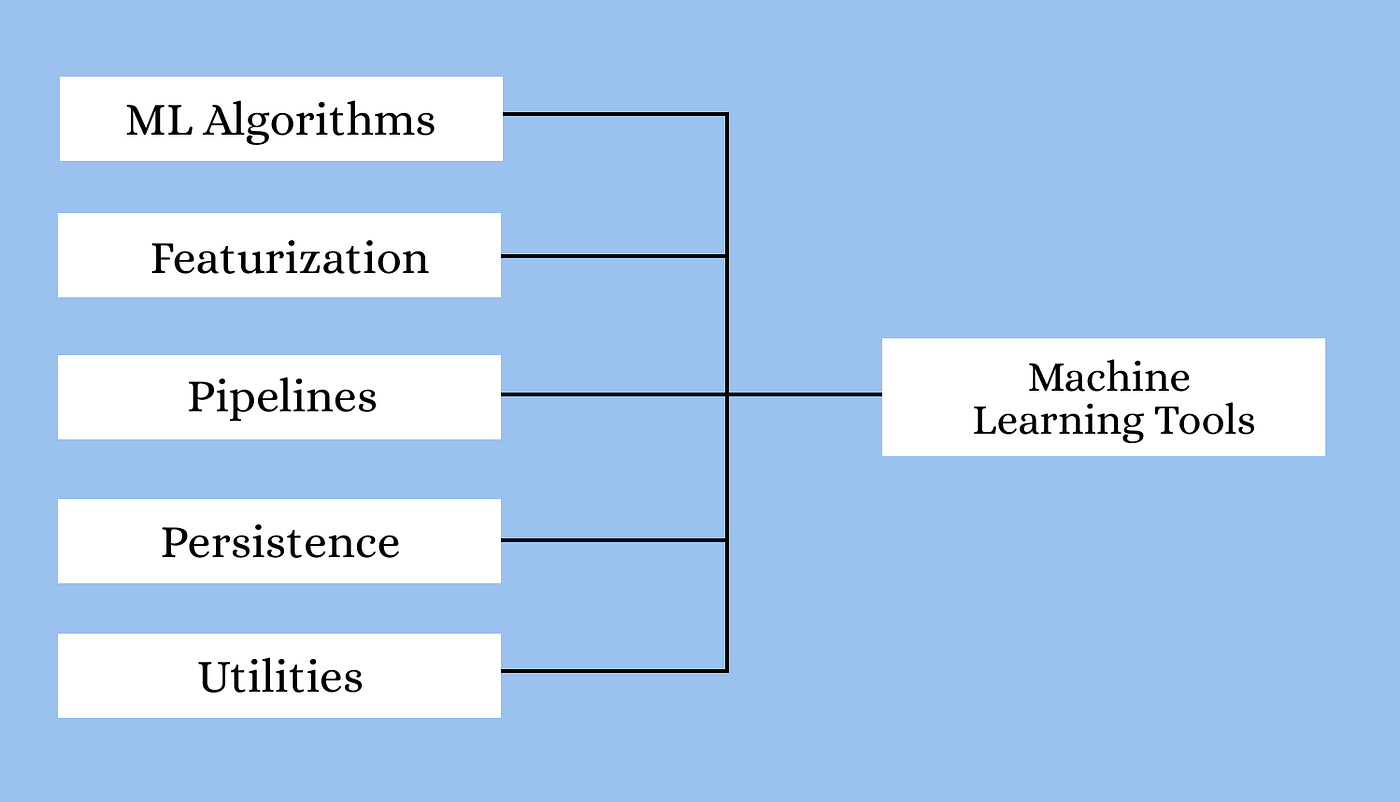Some of the popular algorithms and APIs that programmers working on Machine Learning using Spark MLlib can utilize are:

● Regression

● Clustering

● Optimization

● Dimensional Reduction

● Classification

● Basic Statistics

● Feature Extraction

## TheanoTheano is a powerful Python library enabling easy defining, optimizing and evaluation of powerful mathematical expressions.

We all know that Machine Learning is basically mathematics and statistics. Theano is a popular python library that is used to define, evaluate and optimize mathematical expressions involving multi-dimensional arrays in an efficient manner. It is achieved by optimizing the utilization of CPU and GPU. It is extensively used for unit-testing and self-verification to detect and diagnose different types of errors. Theano is a very powerful library that has been used in large-scale computationally intensive scientific projects for a long time but is simple and approachable enough to be used by individuals for their own projects.

``````# Python program using Theano
# for computing a Logistic
# Function

import theano
import theano.tensor as T
x = T.dmatrix('x')
s = 1 / (1 + T.exp(-x))
logistic = theano.function([x], s)
logistic([[0, 1], [-1, -2]]) ``````

Output:

``````array([[0.5, 0.73105858],
[0.26894142, 0.11920292]])``````

Some of the features that make Theano a robust library for carrying out scientific calculations on a large-scale are:

● Support for GPUs to perform better in heavy-duty computations compared to CPUs

● Strong integration support with NumPy

● Faster and stable evaluations of even the trickiest of variables

● Ability to create custom C code for your mathematical operations

With Theano, you can achieve the rapid development of some of the most efficient machine learning algorithms. Built on top of Theano are some of the well known deep learning libraries such as Keras, Blocks and Lasagne. For more advanced concepts in Theano, you can refer to the Theano tutorial.

## MXNet

A flexible and efficient library for deep learningIf your field of expertise includes Deep Learning, you will find MXNet to be the perfect fit. Used to train and deploy deep neural networks, MXNet is highly scalable and supports quick model training. Apache’s MXNet not only works with Python but also with a host of other languages including C++, Perl, Julia, R, Scala, Go and a few more.

MXNet’s portability and scalability let you take from one platform to another and scale it to the demanding needs of your project. Some of the biggest names in tech and education such as Intel, Microsoft, MIT and more currently support MXNet. Amazon’s AWS prefers MXNet as its choice of preferred deep learning framework.

## SciPySciPy is a very popular library among Machine Learning enthusiasts as it contains different modules for optimization, linear algebra, integration and statistics. There is a difference between the SciPy library and the SciPy stack. The SciPy is one of the core packages that make up the SciPy stack. SciPy is also very useful for image manipulation.

``````# Python script using Scipy
# for image manipulation

from scipy.misc import imread, imsave, imresize

# Read a JPEG image into a numpy array
img = imread('D:/Programs / cat.jpg') # path of the image
print(img.dtype, img.shape)

# Tinting the image
img_tint = img * [1, 0.45, 0.3]

# Saving the tinted image
imsave('D:/Programs / cat_tinted.jpg', img_tint)

# Resizing the tinted image to be 300 x 300 pixels
img_tint_resize = imresize(img_tint, (300, 300))

# Saving the resized tinted image
imsave('D:/Programs / cat_tinted_resized.jpg', img_tint_resize) ``````

Original image:Tinted image:Resized tinted image:## Numpy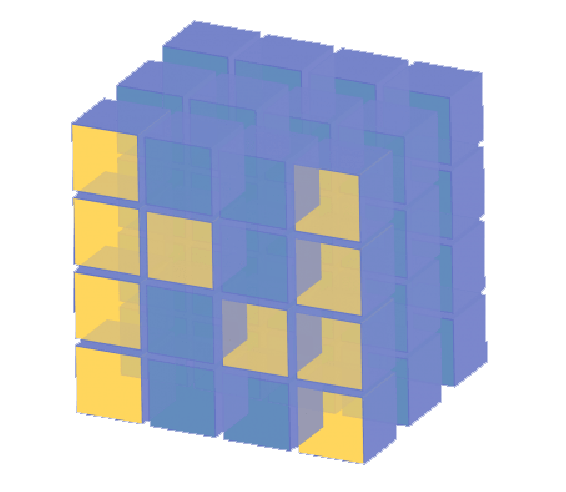The NumPy library for Python concentrates on handling extensive multi-dimensional data and the intricate mathematical functions operating on the data.

NumPy is a very popular python library for large multi-dimensional array and matrix processing, with the help of a large collection of high-level mathematical functions. It is very useful for fundamental scientific computations in Machine Learning. It is particularly useful for linear algebra, Fourier transform, and random number capabilities. High-end libraries like TensorFlow uses NumPy internally for manipulation of Tensors.

``````# Python program using NumPy
# for some basic mathematical
# operations

import numpy as np

# Creating two arrays of rank 2
x = np.array([[1, 2], [3, 4]])
y = np.array([[5, 6], [7, 8]])

# Creating two arrays of rank 1
v = np.array([9, 10])
w = np.array([11, 12])

# Inner product of vectors
print(np.dot(v, w), "\n")

# Matrix and Vector product
print(np.dot(x, v), "\n")

# Matrix and matrix product
print(np.dot(x, y)) ``````

Output:

``````219

[29 67]

[[19 22]
[43 50]]``````

NumPy offers speedy computation and execution of complicated functions working on arrays. Few of the points in favor of NumPy are:

● Support for mathematical and logical operations

● Shape manipulation

● Sorting and Selecting capabilities

● Discrete Fourier transformations

● Basic linear algebra and statistical operations

● Random simulations

● Support for n-dimensional arrays

NumPy works on an object-oriented approach and has tools for integrating C, C++ and Fortran code, and this makes NumPy highly popular amongst the scientific community.

Core task: Data cleaning and manipulation

Google Trends — Numpy Interest Over Time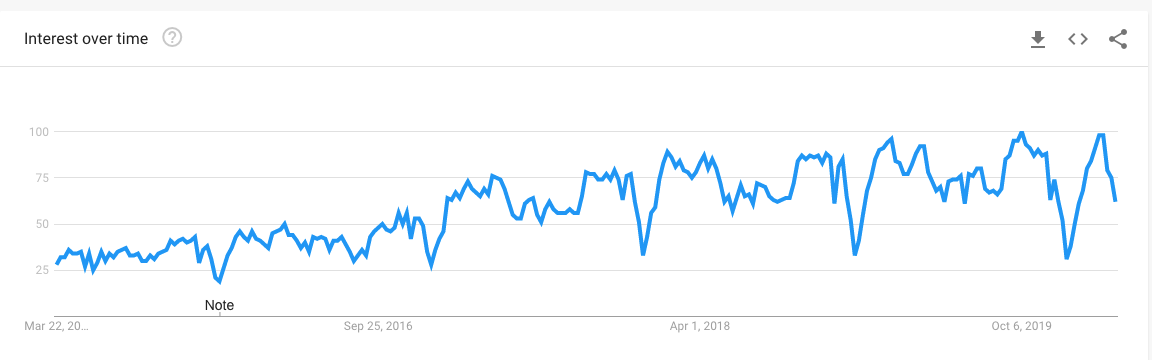## Matplotlib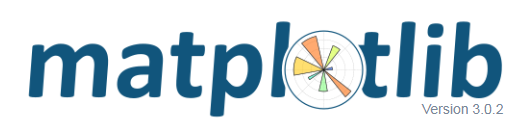Matpoltlib is a very popular Python library for data visualization. Like Pandas, it is not directly related to Machine Learning. It particularly comes in handy when a programmer wants to visualize the patterns in the data. It is a 2D plotting library used for creating 2D graphs and plots. A module named pyplot makes it easy for programmers for plotting as it provides features to control line styles, font properties, formatting axes, etc. It provides various kinds of graphs and plots for data visualization, viz., histogram, error charts, bar chats, etc,

``````# Python program using Matplotib
# for forming a linear plot

# importing the necessary packages and modules
import matplotlib.pyplot as plt
import numpy as np

# Prepare the data
x = np.linspace(0, 10, 100)

# Plot the data
plt.plot(x, x, label ='linear')

plt.legend()

# Show the plot
plt.show() ``````

Output: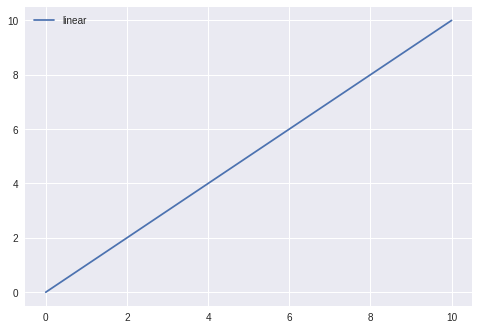For more details refer to documentation.

## Conclusion

Python is a truly marvelous tool of development that not only serves as a general-purpose programming language but also caters to specific niches of your project or workflows. With loads of libraries and packages that expand the capabilities of Python and make it an all-rounder and a perfect fit for anyone looking to get into developing programs and algorithms. With some of the modern machine learning and deep learning libraries for Python discussed briefly above, you can get an idea about what each of these libraries has to offer and make your pick.

## PyTorch for Deep Learning | Data Science | Machine Learning | Python

PyTorch for Deep Learning | Data Science | Machine Learning | Python. PyTorch is a library in Python which provides tools to build deep learning models. What python does for programming PyTorch does for deep learning. Python is a very flexible language for programming and just like python, the PyTorch library provides flexible tools for deep learning.

## Data Augmentation in Deep Learning | Data Science | Machine Learning

Data Augmentation is a technique in Deep Learning which helps in adding value to our base dataset by adding the gathered information from various sources to improve the quality of data of an organisation.

## Machine Learning, Data Science and Deep Learning with Python

Complete hands-on Machine Learning tutorial with Data Science, Tensorflow, Artificial Intelligence, and Neural Networks. Introducing Tensorflow, Using Tensorflow, Introducing Keras, Using Keras, Convolutional Neural Networks (CNNs), Recurrent Neural Networks (RNNs), Learning Deep Learning, Machine Learning with Neural Networks, Deep Learning Tutorial with Python

## PySpark in Machine Learning | Data Science | Machine Learning | Python

PySpark in Machine Learning | Data Science | Machine Learning | Python. PySpark is the API of Python to support the framework of Apache Spark. Apache Spark is the component of Hadoop Ecosystem, which is now getting very popular with the big data frameworks.

## How to get started with Python for Deep Learning and Data Science

A step-by-step guide to setting up Python for Deep Learning and Data Science for a complete beginner